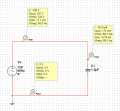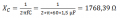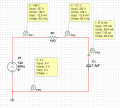# Need help understanding Capacitive Reactance

#### rhille

Joined Feb 29, 2016
7
Hello all

I am trying to understand transformerless PSUs since I want to integrate one in a project I am working on. But before I do that, I am trying to understand the basics like capacitive reactance. For example, I built the following schematic in Multisim:Now, based on the capacitance reactance formula, I get an equivalent of 1768 ohms for C1:By using Ohms' Law, I get for current: I = V/R or 120 / 1768,39 = 0,0679 A or 67,9 mA which is pretty close to what Multisim shows. But still, there is a small difference of just over 1%. Is it normal or am I missing something? No tolerances are set on either parts.

Let's put that aside for a moment and add a resistor to the schematic. This resistor is used to limit current coming into the capacitor.Now, I tried to calculate the current by adding the capacitance reactance of C1 plus the resistor like we usually do for resistors in series (1768 + 1000 ohms) and divide 120V by the total to get the current but I was not able to get the same figures. In fact, I was pretty much unable to get any of the numbers Multisim shows even though I have been searching on the Web for the last few days. I know I am missing something, but for the life of me, I cannot figure out what! How would somebody go about computing all the voltage drops and the current in this circuit? Could somebody please explain to me how to calculate those numbers correctly?

Thanks

Rick

•MrSoftware

#### #12

Joined Nov 30, 2010
18,224
1) Multisim is wrong.
2) (Xc^2 + R^2) = Z^2

#### WBahn

Joined Mar 31, 2012
29,164
The RMS voltage that multisim is calculating is based on the data samples it has for the waveform over some period of time (probably the entire simulation time). So if the simulation time isn't an integer multiple of periods then the RMS (as well as other) values will be off a but.

You need to add the impedances (and the voltages) as phasors, meaning that they not only have a magnitude but also phase shift.

Your simulation gives you the RMS voltage across the capacitor, but only because the node that you have an RMS voltage value for attached to one end of the capacitor is referred to the common node which just happens to be the other end of the capacitor.

You don't have the information for the voltage across the resistor because both of those voltages are referred to a different node (the common node). You can't just subtract them because you don't know the phase shifts.

What we DO know is that, since the two components are in series, that the current through them has the same phase shift in each. We also know that the voltage across a resistor is in phase with the current through it while the voltage across a capacitor lags the current through it by 90°. Thus we know the total voltage across both (which we know is 120 Vrms) will be the Pythagorean sum of the two. Since we know the voltage across the capacitor, we can therefore find the magnitude of the voltage across the resistor.